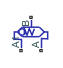# Shuttle Valve (IL)

One-way switching valve in an isothermal liquid system

Since R2020a

•Libraries:
Simscape / Fluids / Isothermal Liquid / Valves & Orifices / Directional Control Valves

## Description

The Shuttle Valve (IL) block models a one-way pressure control valve or switching component in an isothermal liquid network. Flow through the valve travels from port A or port A1 to port B. When the pressure differential between A and A1, pAA1, is above a specified threshold pressure, the path between ports A and B is open to flow and the port at A1 closes. The path has been fully changed when the pressure reaches the Pressure at which A-B is fully open and A1-B is fully closed. When pAA1 falls below the threshold pressure, the flow inlet switches to port A1.

### Mass Flow Rate Equation

Mass is conserved through the valve:

`${\stackrel{˙}{m}}_{A}+{\stackrel{˙}{m}}_{A1}+{\stackrel{˙}{m}}_{B}=0.$`

There is no flow between ports A and A1.

The mass flow rate through the valve is calculated as:

`$\stackrel{˙}{m}=\frac{{C}_{d}{A}_{valve}\sqrt{2\overline{\rho }}}{\sqrt{P{R}_{loss}\left(1-{\left(\frac{{A}_{valve}}{{A}_{port}}\right)}^{2}\right)}}\frac{\Delta p}{{\left[\Delta {p}^{2}+\Delta {p}_{crit}^{2}\right]}^{1/4}},$`

where:

• Cd is the Discharge coefficient.

• Avalve is the valve open area, either between ports A and B or ports A1 and B.

• Aport is the Cross-sectional area at ports A and B.

• $\overline{\rho }$ is the average fluid density.

• Δp is the valve pressure difference. Depending on the flow path through the valve, this is either pApB, pA1pB, or the normalized pressure when switching between the two inlet ports, $\stackrel{^}{p}$, defined below.

The critical pressure difference, Δpcrit, is the pressure differential associated with the Critical Reynolds number, Recrit, and is also dependent on the flow path through the valve:

`$\Delta {p}_{crit}=\frac{\pi \overline{\rho }}{8{A}_{valve}}{\left(\frac{\nu {\mathrm{Re}}_{crit}}{{C}_{d}}\right)}^{2}.$`

The critical Reynolds number is the flow regime transition point between laminar and turbulent flow.

Pressure loss describes the reduction of pressure in the valve due to a decrease in area, and can change if different valve flow paths have different areas. PRloss is calculated as:

`$P{R}_{loss}=\frac{\sqrt{1-{\left(\frac{{A}_{valve}}{{A}_{port}}\right)}^{2}\left(1-{C}_{d}^{2}\right)}-{C}_{d}\frac{{A}_{valve}}{{A}_{port}}}{\sqrt{1-{\left(\frac{{A}_{valve}}{{A}_{port}}\right)}^{2}\left(1-{C}_{d}^{2}\right)}+{C}_{d}\frac{{A}_{valve}}{{A}_{port}}}.$`

Pressure recovery describes the positive pressure change in the valve due to an increase in area. If you do not wish to capture this increase in pressure, set the Pressure recovery to `Off`. In this case, PRloss is 1.

The opening area, Avalve, is also impacted by the valve opening dynamics.

### Opening Parameterization

The linear parameterization of the valve area depends on the flow path through the valve. The dynamic area is based on a normally open path between ports A and B:

`${A}_{valve,AB}=\stackrel{^}{p}\left({A}_{\mathrm{max}}-{A}_{leak}\right)+{A}_{leak}.$`

The normalized pressure, $\stackrel{^}{p}$, is

`$\stackrel{^}{p}=\frac{{p}_{AA1}-{p}_{open,A1B}}{{p}_{open,AB}-{p}_{open,A1B}},$`

when pAA1 is between the parameters Pressure at which A-B is fully closed and A1-B is fully open and Pressure at which A-B is fully open and A1-B is fully closed. If pAA1 is below Pressure at which A-B is fully closed and A1-B is fully open, $\stackrel{^}{p}$ is 0. If pAA1 is above Pressure at which A-B is fully open and A1-B is fully closed, $\stackrel{^}{p}$ is 1.

When the valve inlet switches from port A to port A1, the valve opening area is:

`${A}_{valve,A1B}={A}_{\mathrm{max}}+{A}_{leak}-{A}_{valve,AB}.$`

When the valve is in a near-open or near-closed position in the linear parameterization, you can maintain numerical robustness in your simulation by adjusting the parameter. If the parameter is nonzero, the block smoothly saturates the normalized control pressure between `0` and `1`. For more information, see Numerical Smoothing.

### Opening Dynamics

If opening dynamics are modeled, a lag is introduced to the flow response to the modeled control pressure. pcontrol becomes the dynamic control pressure, pdyn; otherwise, pcontrol is the steady-state pressure. The instantaneous change in dynamic control pressure is calculated based on the Opening time constant, τ:

`${\stackrel{˙}{p}}_{dyn,AB}=\frac{{p}_{control}-{p}_{dyn,AB}}{\tau }.$`

By default, Opening dynamics is set to `Off`.

## Ports

### Conserving

expand all

Entry port to the valve.

Entry port to the valve.

Exit port from the valve.

## Parameters

expand all

Pressure threshold between ports A and A1, below which the flow path between ports A1 and B is fully open and the flow path between ports A and B is fully closed.

Pressure threshold between ports A and A1, above which the flow path between ports A and B is fully open and the flow path between ports A1 and B is fully closed.

Maximum open area of the valve. This value is used to determine the normalized valve pressure and the valve opening area during operation.

Sum of all gaps when the valve is in the fully closed position. Any area smaller than this value is saturated to the specified leakage area. This contributes to numerical stability by maintaining continuity in the flow.

Areas at the entry and exit ports A, A1, and B, which are used in the pressure-flow rate equation that determines the mass flow rate through the valve.

Correction factor accounting for discharge losses in theoretical flows.

Upper Reynolds number limit for laminar flow through the orifice.

Continuous smoothing factor that introduces a layer of gradual change to the flow response when the valve is in near-open or near-closed positions. Set this value to a nonzero value less than one to increase the stability of your simulation in these regimes.

Whether to account for pressure increase when fluid flows from a region of smaller cross-sectional area to a region of larger cross-sectional area.

Whether to account for transient effects to the fluid system due to opening the valve. Setting Opening dynamics to `On` approximates the opening conditions by introducing a first-order lag in the flow response. The Opening time constant also impacts the modeled opening dynamics.

Constant that captures the time required for the fluid to reach steady-state conditions when opening or closing the valve from one position to another. This parameter impacts the modeled opening dynamics.

#### Dependencies

To enable this parameter, set Opening dynamics to `On`.

## Version History

Introduced in R2020a